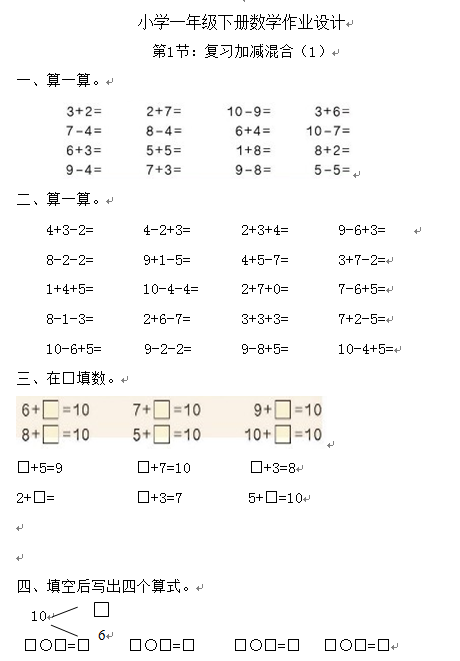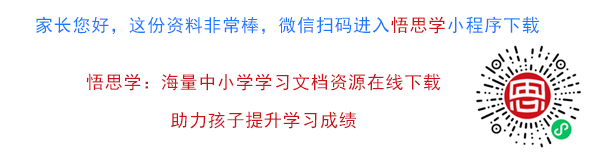• ：复习加减混合（1）
• 算一算。

4+3-2=       4-2+3=       2+3+4=       9-6+3=

8-2-2=       9+1-5=       4+5-7=       3+7-2=

【DOC文档63页】小学新人教版一年级下册数学作业题 A4电子版资料_可直接打印_会员免费下载##### 评论信息